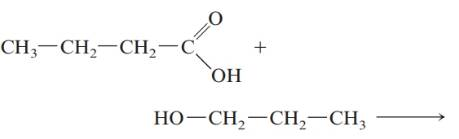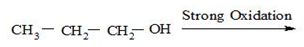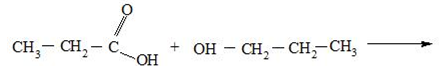Chapter 20, Problem 78QAP### Introductory Chemistry: A Foundati...

9th Edition
Steven S. Zumdahl + 1 other
ISBN: 9781337399425

#### Solutions

Chapter
Section### Introductory Chemistry: A Foundati...

9th Edition
Steven S. Zumdahl + 1 other
ISBN: 9781337399425
Textbook Problem
1 views

# . Complete the following equations with the structural formula of the principal organic product.a. CH 3 — CH 2 — CH 2 — CH 2 — OH → Mild oxidation    b. CH 3 — CH 2 — CH 2 — OH → Strong oxidation cInterpretation Introduction

(a)

Interpretation:The principal organic product of the following reaction should be determined:

Concept Introduction:

Alcohols are organic compounds which have terminal hydroxyl (OH) groups which can oxidize into aldehydes or ketones by mild oxidation.

Explanation

This is a primary a...

Interpretation Introduction

(b)

Interpretation:

The principal organic product of the following reaction should be determined:Concept Introduction:

Alcohols are organic compounds which have terminal hydroxyl (OH) groups which can oxidize into carboxylic acids by strong oxidation.

Interpretation Introduction

(c)

Interpretation:

The principal organic product of the following reaction should be determined:Concept Introduction:

Alcohols are organic compounds which have terminal hydroxyl (OH) groups while carboxyl acids have carboxyl groups ( COOH ). Ester and a water molecule is the result of a reaction between an alcohol and carboxylic acid.

### Still sussing out bartleby?

Check out a sample textbook solution.

See a sample solution

#### The Solution to Your Study Problems

Bartleby provides explanations to thousands of textbook problems written by our experts, many with advanced degrees!

Get Started